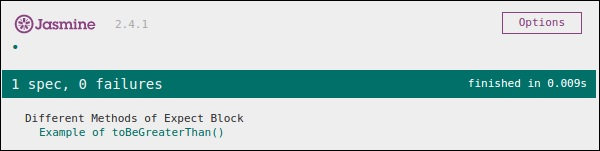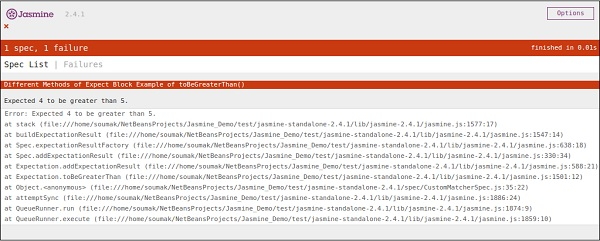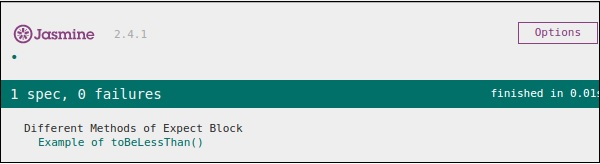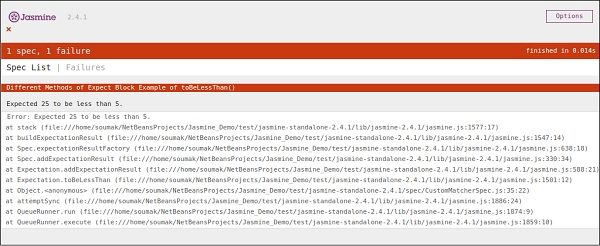# JasmineJS - Inequality Check

Till now, we have discussed different methods in Jasmine which help us test different scenarios based on our requirements. In this chapter, we will learn about different matchers that will help us check the inequality condition in JS file. Following are the matchers used for this purpose.

## ToBeGreaterThan()

As the name suggests this matcher helps to check greater than condition. Let us modify our customerMatcher.js using the following piece of code.

```describe("Different Methods of Expect Block",function () {
var exp = 8;

it("Example of  toBeGreaterThan()", function () {
expect(exp).toBeGreaterThan(5);
});
});
```

In the above piece of code, we are expecting that the value of the variable “exp” will be greater than 5. Now as the value of the variable “exp” is “8” which is greater than “5”, this piece of code will generate a green screenshot.Now again let us modify the value of the variable to “4” and make this test fail. To do that we need to modify the js file using the following piece of code.

```describe("Different Methods of Expect Block",function () {
var exp = 4;

it ("Example of toBeGreaterThan()", function () {
expect(exp).toBeGreaterThan(5);
});
});
```

This code will fail because value 4 cannot be greater than 5. Hence it will produce the following output.## ToBeLessThan()

This matcher helps to check the less than condition of the test scenario. It behaves exactly opposite to that of toBeGreaterThan() matcher. Now let us see how this matcher works. Let us modify the customerMatcher.js file accordingly.

```describe("Different Methodsof Expect Block",function () {
var exp = 4;

it("Example of toBeLessThan()", function() {
expect(exp).toBeLessThan(5);
});
});
```

Like the previous example, we have one variable having value as “4”. In this piece of code, we are checking whether the value of this variable is less than 5 or not. This piece of code will generate the following output.Now to make this fail, we need to assign some bigger number to the variable exp. Let us do that and test the application. We will assign 25 as the value to the exp, which will definitely throw an error and yield the following screenshot in red.